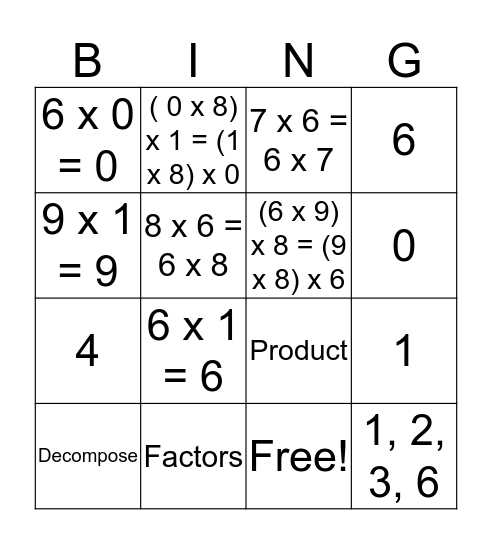# Multiplication Property BingoThis bingo card has 17 words: Free!, Decompose, 7 x 6 = 6 x 7, 6 x 0 = 0, 6 x 1 = 6, 6, 1, (6 x 9) x 8 = (9 x 8) x 6, 0, 8 x 6 = 6 x 8, Factors, Product, 9 x 1 = 9, ( 0 x 8) x 1 = (1 x 8) x 0, 4, 9 and 1, 2, 3, 6.

## Play Online